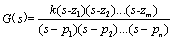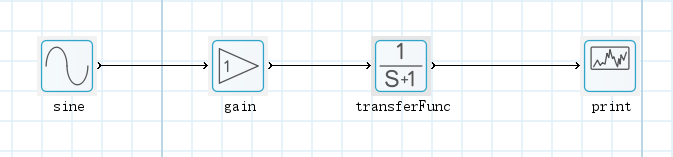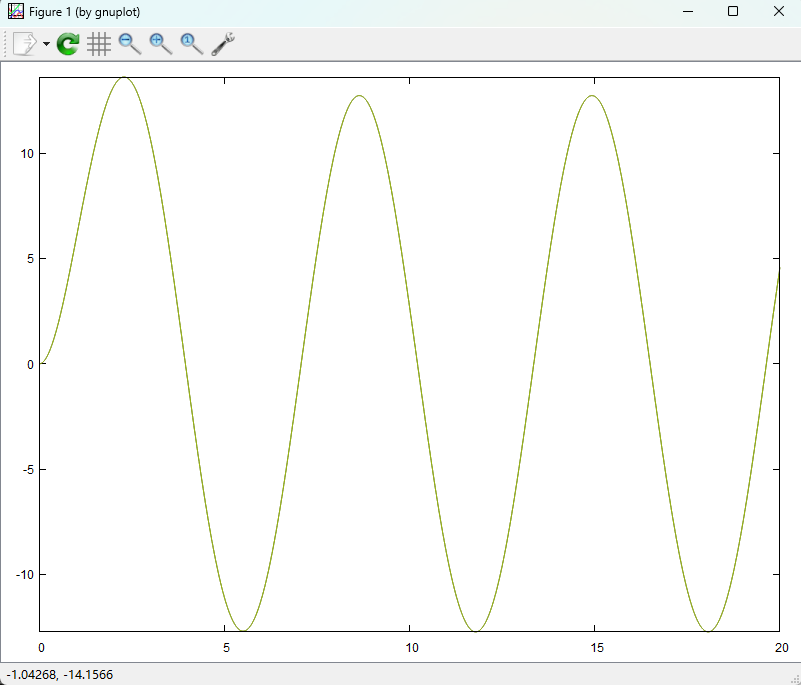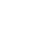ZPG = zpk(z, p, k)

G(s) = 18(s + 2) / (s + 0.4)(s + 15)(s + 25)

G(s) = 18(s + 2)/(s^3 + 40.4s^2 + 391s + 150)204 0 0 收藏 回复

## 回复

### 社区牛人